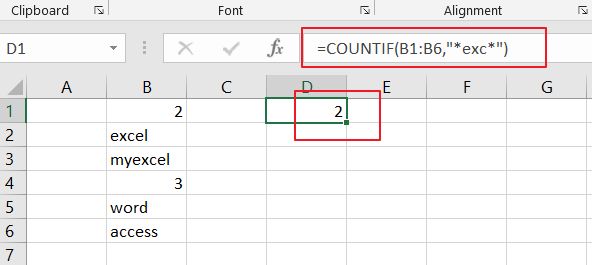# How to Count Cells that Contain Specific Text in Excel

This post will guide you how to count the number of cells that specific text within a range of cells using a formula in Excel 2013/2016 or Excel office 365. How do I count the number of cells with certain text using a simple formula in Excel. When you analyze a large list of data, you may also want to know that how many cells that contain specific text. The below steps will show you how to do it in a simple way.

## Count Number of Cells that Contain Specific Text

You can use the COUNTIF function to count cells that contain certain text. And you also need to supply the target text string in the criteria argument in the COUNTIF function.

The below is a generic formula to count the number of cells containing specific text:

=COUNTIF(range,criteria)

Assuming that you have a list of data in range B1:B6 and you want to count the number of cells with a certain text “exc”. And you can type this string in the second argument as its criteria and you will get the below formula:

=COUNTIF(B1:B6,”*exc*”)LET’S SEE THAT HOW THIS FORMULA WORKS:

The COUNTIF function can be used to count the number of cells that match a single condition or criteria. And in this case, you need to provide a criteria as “*exc*”, which is evaluated as “values that containing ‘exc’ in any position”. Then the total count of all cells in the range B1:B6 that meet the above criteria is returned.

Note: the asterisk(*) means that it will match any number of characters in the above formula. If you just only supply a certain string “exc”, it means that cells must contain exactly “exc”. If the cell contains any other characters, it will not be counted. So you need to use the asterisk character as a wildcard in the second criteria argument.

### Related Functions

• Excel COUNTIF function
The Excel COUNTIF function will count the number of cells in a range that meet a given criteria. This function can be used to count the different kinds of cells with number, date, text values, blank, non-blanks, or containing specific characters. etc. = COUNTIF (range, criteria) …